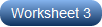1. Worksheets>
2. Math>
3. Trigonometry>
4. Allied Angles

# Trigonometric Ratios of Allied Angles

Allied angles worksheets, designed specifically for high school students feature topics like finding the exact value of the trigonometric ratio offering angle measures in degrees or radians, evaluating trig ratios of allied angles and proving the trigonometric statements to mention just a few. Precede your practice with a thorough knowledge of quadrants, degrees and radians from the ASTC chart included here. Try some of these worksheets for free!

Find the Exact Value: (Degrees)

Explore the printable worksheets here containing skills to find the value of trigonometric ratios of angles presented in the form of degrees. Make use of the allied angle chart in degrees to complete the exercises.Related Worksheets